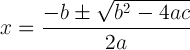×

# Quadratic Formula Calculator

## Enter the coefficients for the quadratic equation ax2 + bx + c = 0:

 a: b: c:

The online Quadratic Formula Calculator is used to solve a quadratic equation using the quadratic formula.

In mathematics, a quadratic equation is a polynomial equation of the second degree. The general form of a quadratic equation is:

ax2 + bx + c = 0

where x represents a variable, and a, b, and c are constants with a ≠ 0.